## 加速度计和陀螺指南

2018年03月19日 ⁄ 综合 ⁄ 共 12535字 ⁄ 字号 评论关闭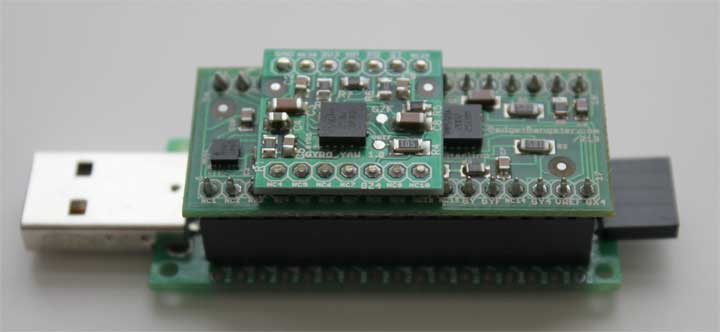2012-8-22 16:38 上传

IMU单元例子：上图中MCU顶端的ACC Gyro 6DOF，名为USBThumb，支持USB/串口通信

- 加速度计（accelerometer）检测什么
- 陀螺仪（gyroscope，也称作 gyro）检测什么
- 如何结合加速度传感器和陀螺仪的数据以得到设备和地平面之间的倾角的准确信息

- LIS331AL (datasheet) – 3轴 2G 模拟加速度计
- LPR550AL (datasheet) – 双轴（俯仰、翻滚） 500°/s 加速度传感器
- LY550ALH (datasheet) –单轴（偏航）陀螺仪 最后这个设备在这篇介绍中不使用，不过他在 DCM
Matrix implementation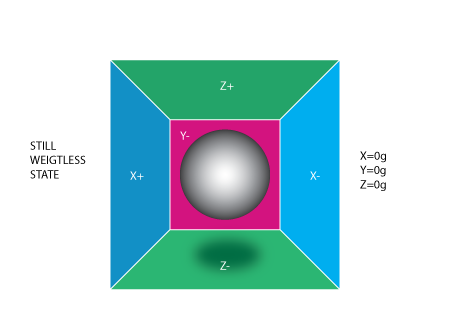2012-8-22 16:38 上传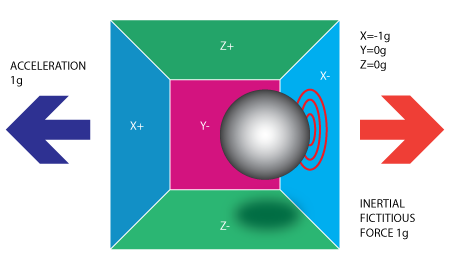2012-8-22 16:38 上传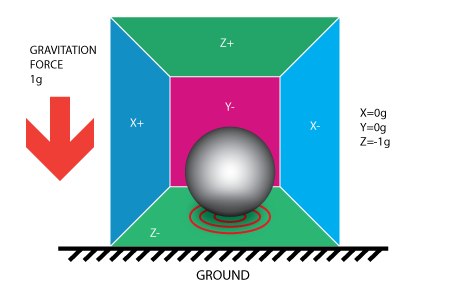2012-8-22 16:38 上传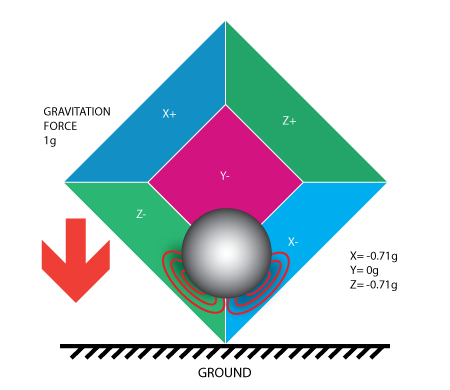2012-8-22 16:38 上传

0.71g这个值是不是任意的，它们实际上是1/2的平方根的近似值。我们介绍加速度计的下一个模型时这一点会更清楚。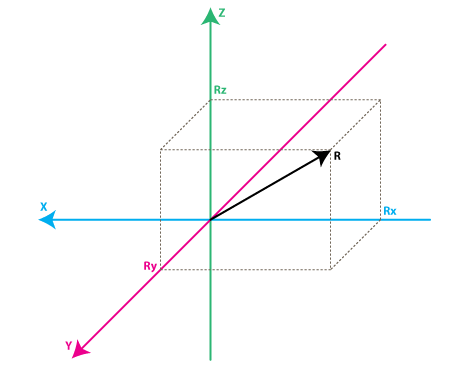2012-8-22 16:38 上传

，R ^ 2 = RX ^ 2 + RY ^ 2 + RZ ^ 2（公式1

1 ^ 2 =（SQRT（1/2））^ 2 + 0 ^ 2 +（SQRT（1/2））^ 2

.. 1023间，请注意，1023 = 2 ^ 10 -1。一个12位ADC模块的输出值范围在0 .. 4095内，注意，4095 = 2 ^ 12-1。

VoltsRx = AdcRx * VREF / 1023

VoltsRx = 586 * 3.3 / 1023 =～1.89V（结果取两位小数）
VoltsRy = 630 * 3.3 / 1023 =～2.03V
VoltsRz = 561 * 3.3 / 1023 =～1.81V

DeltaVoltsRx = 1.89V - 1.65V = 0.24V
DeltaVoltsRy = 2.03V - 1.65V = 0.38V
DeltaVoltsRz = 1.81V - 1.65V = 0.16V

RX = DeltaVoltsRx /Sensitivity
RX = 0.24V / 0.4785V / G =～0.5g
RY = 0.38V / 0.4785V / G =～0.79g
RZ = 0.16V / 0.4785V / G =～0.33g

Rx = (AdcRx * Vref / 1023 – VzeroG) / Sensitivity (公式2)
Ry = (AdcRy * Vref / 1023 – VzeroG) / Sensitivity
Rz = (AdcRz * Vref / 1023 – VzeroG) / Sensitivity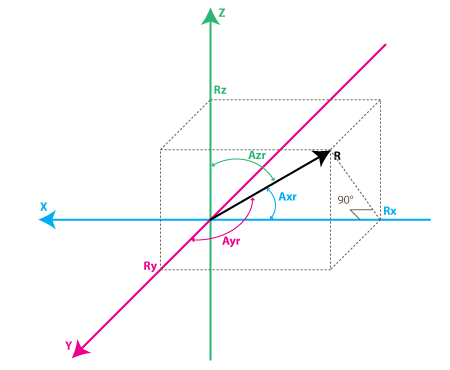2012-8-22 16:38 上传

cos(Axr) = Rx / R , 类似的：
cos(Ayr) = Ry / R
cos(Azr) = Rz / R

Axr = arccos(Rx/R)
Ayr = arccos(Ry/R)
Azr = arccos(Rz/R)

cosX = cos(Axr) = Rx / R
cosY = cos(Ayr) = Ry / R
cosZ = cos(Azr) = Rz / R

SQRT（cosX ^ 2 + COSY ^ 2 + cosZ ^ 2）= 1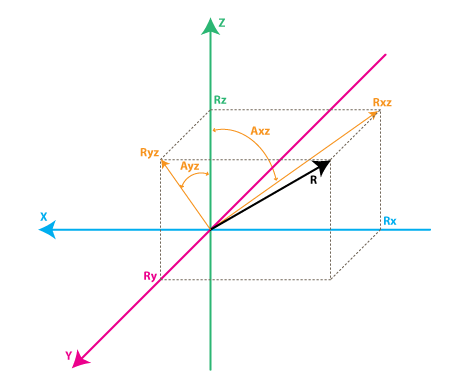2012-8-22 16:38 上传

Rxz – 惯性力矢量R在XZ平面上的投影
Ryz – 惯性力矢量R在YZ平面的上投影

Rxz^2 = Rx^2 + Rz^2 ，同样：
Ryz^2 = Ry^2 + Rz^2

R^2 = Rxz^2 + Ry^2 ，这个公式可以公式1和上面的公式推导出来，也可由R和Ryz所组成的直角三角形推导出来
R ^ 2 = Ryz ^ 2 + RX ^ 2

AXZ - Rxz（矢量R在XZ平面的投影）和Z轴所成的夹角
AYZ - Ryz（矢量R在YZ平面的投影）和Z轴所成夹角

RateAxz = (Axz1 – Axz0) / (t1 – t0).

RateAxz = (AdcGyroXZ * Vref / 1023 – VzeroRate) / Sensitivity 公式3
RateAyz = (AdcGyroYZ * Vref / 1023 – VzeroRate) / Sensitivity
VzeroRate – 是零变化率电压，换句话说它是陀螺仪不受任何转动影响时的输出值，对Acc Gyro板来说，可以认为是1.23V（此值通常可以在说明书中找到——但千万别相信这个值，因为大多数的陀螺仪在焊接后会有一定的偏差，所以可以使用电压计测量每个通道的输出值，通常这个值在焊接后就不会改变，如果有跳动，在设备使用前写一个校准程序对其进行测量，用户应当在设备启动的时候保持设备静止以进行校准）。
Sensitivity –陀螺仪的灵敏度，单位mV/(deg/s)，通常写作mV/deg/s,它的意思就是如果旋转速度增加1°/s,陀螺仪的输出就会增加多少mV。Acc_Gyro板的灵敏度值是2mV/deg/s或0.002V/deg/s

RateAxz = (571 * 3.3V / 1023 – 1.23V) / ( 0.002V/deg/s) =~ 306 deg/s
RateAyz = (323 * 3.3V / 1023 – 1.23V) / ( 0.002V/deg/s) =~ -94 deg/s

http://starlino.com/imu_kalman_arduino.html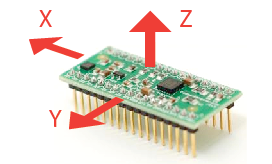2012-8-22 16:39 上传

- 确定陀螺仪的输出对应到上述讨论的RateAxz，RateAyz值。
- 根据陀螺仪和加速度计的位置决定是否要反转输出值

- 首先将设备保持水平。加速度计的XY轴输出会是零加速度电压（Acc Gyro板的值是1.65V）
- 接下来将设备绕Y轴旋转，换句话说就是将设备在XZ平面内旋转，所以X、Z的加速度输出值会变化而Y轴保持不变。
-当以匀速旋转设备的时候，注意陀螺仪的哪个通道输出值变化了，其他输出应该保持不变。
-最后一步，确认旋转的方向是否和我们的模型对应，因为陀螺仪和加速度的位置关系，有时候你可能要把RateAxz值反向
RateAxz = InvertAxz * (AdcGyroXZ * Vref / 1023 – VzeroRate) / Sensitivity ,其中InvertAxz= 1 或-1

RateAyz = InvertAyz * (AdcGyroYZ * Vref / 1023 – VzeroRate) / Sensitivity

- RateAxz的输出管脚是GX4，InvertAxz = 1
- RateAyz输出管脚是GY4，InvertAyz = 1

Racc – 是由加速度计测量到得惯性力矢量，它可分解为下面的分量（在XYZ轴上的投影）：
RxAcc = (AdcRx * Vref / 1023 – VzeroG) / Sensitivity
RyAcc = (AdcRy * Vref / 1023 – VzeroG) / Sensitivity
RzAcc = (AdcRz * Vref / 1023 – VzeroG) / Sensitivity

Racc = [RxAcc,RyAcc,RzAcc]

|Racc| = SQRT(RxAcc^2 +RyAcc^2 + RzAcc^2),

Racc(normalized) = [RxAcc/|Racc| , RyAcc/|Racc| , RzAcc/|Racc|].

Rest = [RxEst,RyEst,RzEst]

-加速度计告诉我们：“你现在的位置是Racc”

-然后根据陀螺仪的数据和上一次的Rest值修正这个值并输出新的估算值Rest。
-我们认为Rest是当前设备姿态的“最佳值”。

Rest(0) = Racc(0)
Rest和Racc是向量，所以上面的式子可以用3个简单的式子代替，注意别重复了：
RxEst（0）= RxAcc（0）
RyEst（0）= RyAcc（0）
RzEst（0）= RzAcc（0）

Rest(n-1) – 前一个估算值，Rest(0) = Racc(0)
Racc(n) – 当前加速度计测量值

Rgyro = [RxGyro,RyGyro,RzGyro]2012-8-22 16:39 上传

tan(Axz) = Rx/Rz => Axz = atan2(Rx,Rz)

Axz(n-1) = atan2( RxEst(n-1) , RzEst(n-1) ).

Axz(n) = Axz(n-1) + RateAxz(n) * T

RateAxzAvg =（RateAxz（N）+ RateAxz（N-1））/ 2
Axz(n) = Axz(n-1) + RateAxzAvg * T

Ayz(n) = Ayz(n-1) + RateAyz(n) * T

| Rgyro | = SQRT（RxGyro ^ 2 + RyGyro ^ 2 + RzGyro ^ 2）

| Rgyro | = 1

x =RxGyro , y=RyGyro, z=RzGyro

x = x / 1 = x / SQRT(x^2+y^2+z^2)

x = ( x / SQRT(x^2 + z^2) ) / SQRT( (x^2 + y^2 + z^2) / (x^2 + z^2) )

x = sin(Axz) / SQRT (1 + y^2 / (x^2 + z^2) )

x = sin(Axz) / SQRT (1 + y^2  * z ^2 / (z^2 * (x^2 + z^2)) )

x = sin(Axz) / SQRT (1 + cos(Axz)^2 * tan(Ayz)^2 )

RxGyro = sin(Axz(n)) / SQRT (1 + cos(Axz(n))^2 * tan(Ayz(n))^2 )

RyGyro = sin(Ayz(n)) / SQRT (1 + cos(Ayz(n))^2 * tan(Axz(n))^2 )

RxGyro =  1  / SQRT (1/ sin(Axz(n))^2  + cos(Axz(n))^2 / sin(Axz(n))^2  * tan(Ayz(n))^2 )
RxGyro =  1  / SQRT (1/ sin(Axz(n))^2  + cot(Axz(n))^2  * sin(Ayz(n))^2  / cos(Ayz(n))^2 )

RxGyro =  1  / SQRT (1/ sin(Axz(n))^2  -  cos(Axz(n))^2/sin(Axz(n))^2   + cot(Axz(n))^2  * sin(Ayz(n))^2  / cos(Ayz(n))^2  + cot(Axz(n))^2 )

RxGyro =  1  / SQRT (1  +   cot(Axz(n))^2 * sec(Ayz(n))^2 ),     其中  cot(x) = 1 / tan(x)  ， sec(x) = 1 / cos(x)

RzGyro  =  Sign(RzGyro)*SQRT(1 – RxGyro^2 – RyGyro^2).

Sign(RzGyro) = Sign(RzEst(n-1))

Racc – 加速度计读取的当前值
Rgyro –根据Rest(-1)和当前陀螺仪读取值所得

Rest(n) = (Racc * w1 + Rgyro * w2 ) / (w1 + w2)

Rest(n) = (Racc * w1/w1 + Rgyro * w2/w1 ) / (w1/w1 + w2/w1)

Rest(n) = (Racc + Rgyro * wGyro ) / (1 + wGyro)

RxEst(n) = (RxAcc + RxGyro * wGyro ) / (1 + wGyro)
RyEst(n) = (RyAcc + RyGyro * wGyro ) / (1 + wGyro)
RzEst(n) = (RzAcc + RzGyro * wGyro ) / (1 + wGyro)

R = SQRT(RxEst(n) ^2 + RyEst(n)^2 +  RzEst(n)^2 )
RxEst(n) = RxEst(n)/R
RyEst(n) = RyEst(n)/R
RzEst(n) = RzEst(n)/R

http://starlino.com/imu_kalman_arduino.html